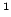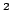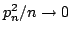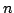Statistica Sinica 21 (2011), 391-419

NONCONCAVE PENALIZED M-ESTIMATION WITH

A DIVERGING NUMBER OF PARAMETERS

Gaorong Li, Heng Pengand Lixing ZhuBeijing University of Technology andHong Kong Baptist University

Abstract: M-estimation is a widely used technique for robust statistical inference. In this paper, we investigate the asymptotic properties of a nonconcave penalized M-estimator in sparse, high-dimensional, linear regression models. Compared with classic M-estimation, the nonconcave penalized M-estimation method can perform parameter estimation and variable selection simultaneously. The proposed method is resistant to heavy-tailed errors or outliers in the response. We show that, under certain appropriate conditions, the nonconcave penalized M-estimator has the so-called Oracle Property"; it is able to select variables consistently, and the estimators of nonzero coefficients have the same asymptotic distribution as they would if the zero coefficients were known in advance. We obtain consistency and asymptotic normality of the estimators when the dimensionof the predictors satisfies the conditionsand, respectively, whereis the sample size. Based on the idea of sure independence screening (SIS) and rank correlation, a robust rank SIS (RSIS) is introduced to deal with ultra-high dimensional data. Simulation studies were carried out to assess the performance of the proposed method for finite-sample cases, and a dataset was analyzed for illustration.

Key words and phrases: Linear model, oracle property, rank correlation, robust estimation, SIS, variable selection.